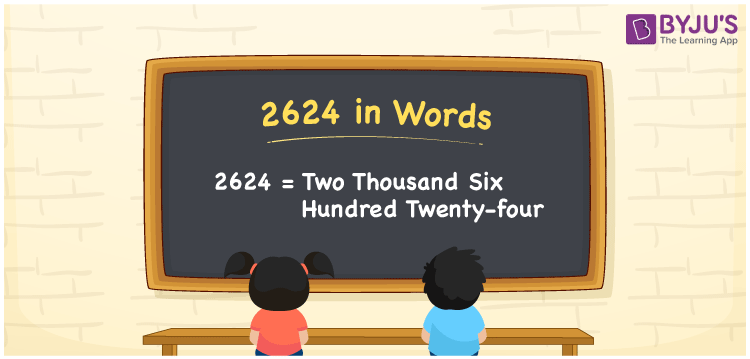# 2624 in Words

We can write 2624 in words as Two Thousand Six Hundred Twenty-four. Suppose you have Rs. 2624 in your bank account, you can say that “I have Two Thousand Six Hundred Twenty-four rupees in my bank account”. It is possible to convert 2624 into words with the help of a place value chart. Also, we know that 2624 is a cardinal number since it represents a specific quantity.

 2624 in words Two Thousand Six Hundred Twenty-four Two Thousand Six Hundred Twenty-four in Numbers 2624

## 2624 in English Words## How to Write 2624 in Words?

We can convert 2624 to words using a place value chart. This can be done as follows. The number 2624 has 4 digits, so let’s make a chart that shows the place value up to 4 digits.

 Thousands Hundreds Tens Ones 2 6 2 4

Thus, we can write the expanded form as:

2 × Thousand + 6 × Hundred + 2 × Ten + 4 × One

= 2 × 1000 + 6 × 100 + 2 × 10 + 4 × 1

= 2624

= Two Thousand Six Hundred Twenty-four

2624 is the natural number that is succeeded by 2623 and preceded by 2625.

2624 in words – Two Thousand Six Hundred Twenty-four

Is 2624 an odd number? – No.

Is 2624 an even number? – Yes

Is 2624 a perfect square number? – No

Is 2624 a perfect cube number? – No

Is 2624 a prime number? – No

Is 2624 a composite number? – Yes

## Frequently Asked Questions on 2624 in Words

Q1

### How to write 2624 in words?

2624 in English is written as “Two Thousand Six Hundred Twenty-four”.
Q2

### Is 2624 a prime number?

No, the number 2624 is not a prime number. Two Thousand Six Hundred Twenty-four is a composite number. Because 2624 has more divisors than 1 and itself.
Q3

### Write Two Thousand Six Hundred Twenty-four in numbers.

Two Thousand Six Hundred Twenty-four in numbers is 2624.